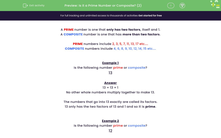# Is it a Prime Number or Composite? (2)

In this worksheet, students decide whether or not a given number is prime or composite.Key stage:  KS 2

Curriculum topic:   Number: Multiplication and Division

Curriculum subtopic:   Use Correct Vocabulary

Difficulty level:#### Worksheet Overview

A PRIME number is one that only has two factors, itself and 1.

A COMPOSITE number is one that has more than two factors.

PRIME numbers include 2, 3, 5, 7, 11, 13, 17 etc....

COMPOSITE numbers include 4, 6, 8, 9, 10, 12, 14, 15 etc....

Example 1

Is the following number prime or composite?

13

13 = 13 × 1

No other whole numbers multiply together to make 13.

The numbers that go into 13 exactly are called its factors.

13 only has the two factors of 13 and 1 and so it is prime.

Example 2

Is the following number prime or composite?

12

12 = 12 × 1

12 = 6 × 2

12 = 4 × 3

The numbers that go into 12 exactly are called its factors.

12 has factors of 12, 6, 4, 3, 2 and 1 and so it is composite.

### What is EdPlace?

We're your National Curriculum aligned online education content provider helping each child succeed in English, maths and science from year 1 to GCSE. With an EdPlace account you’ll be able to track and measure progress, helping each child achieve their best. We build confidence and attainment by personalising each child’s learning at a level that suits them.

Get started